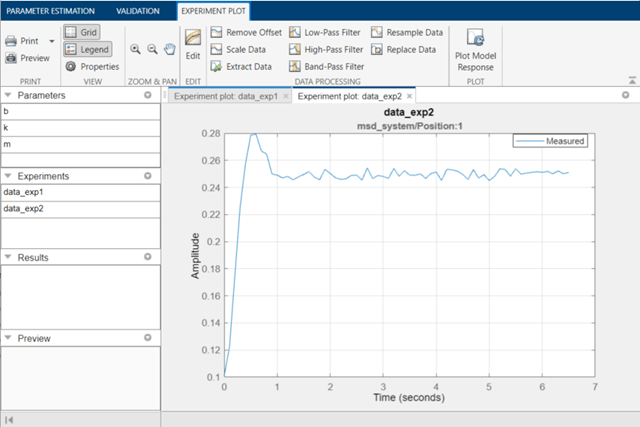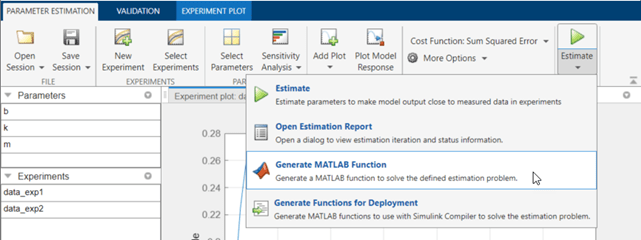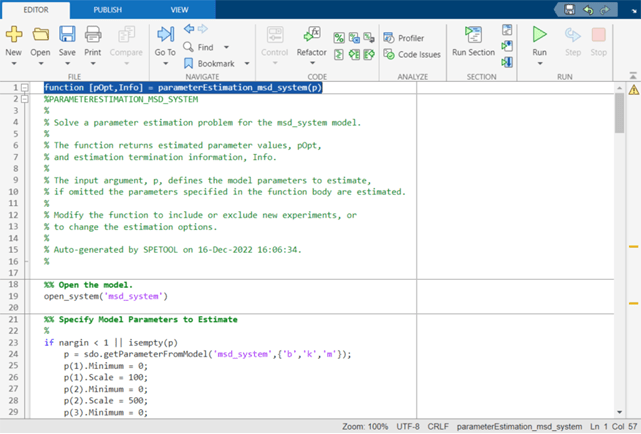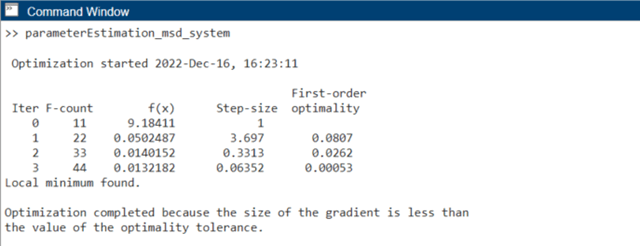Generate MATLAB Code for Parameter Estimation Problems (GUI)

This example shows how to automatically generate a MATLAB® function to solve a parameter estimation problem. You use the Parameter Estimator to define an estimation problem for a mass-spring-damper and generate MATLAB code to solve this estimation problem.

Mass-Spring-Damper Estimation Problem

The Estimate Model Parameters and Initial States (GUI) example shows how to use the Parameter Estimator to estimate parameters of a mass-spring-damper model. In this example, you load a pre-configured Parameter Estimator session based on that example.

Use the following commands to load the pre-configured estimation session.

spetool(SDOSessionData)Generate MATLAB Code

From the Estimate list, select Generate MATLAB Code.The generated code is added to the MATLAB editor as an unsaved MATLAB function.Examine the generated code. Significant code portions are:

• Specify Model Parameters to Estimate - Definition of the model parameters being estimated.

• Define the Estimation Experiments - Definition of the measured and expected signal data to use for estimation.

• Create Estimation Objective Function - Creation of an anonymous function that calls the subfunction msd_system_optFcn, which evaluates the model using each experiment and compares simulation and measured experiment outputs. This anonymous function is called by sdo.optimize at each iteration of the optimization problem to solve the estimation problem.

• Estimate the Parameters - Solve the estimation problem using the sdo.optimize command.

Select Save from the MATLAB editor to save the generated function.

Run Generated Code

Run the generated function.The first output argument, pOpt, contains the optimized parameter values and the second output argument, optInfo, contains optimization information.

Modify the Generated Code

You can:

• Modify the generated spe_msd_system function to include or exclude new experiments or change estimation options.

• Call the generated spe_msd_system function with a different set of parameters to estimate.

For details on how to write an objective/constraint function to use with the sdo.optimize command, type help sdoExampleCostFunction at the MATLAB command prompt.

Close the model.